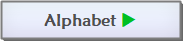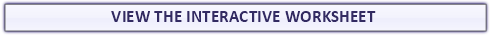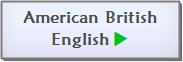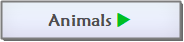Found a mistake?
!## Number 1 Kindergarten Tracing Exercise Maths Worksheet

A fun printable maths number tracing exercise worksheet with elephant theme to teach kindergarten, nursery school and preschool kids and students how to write the number 1 (one) and how to count. Look at the samples. Follow the traces and practise writing the number 1. Then color the elephant.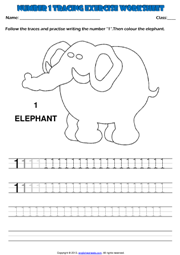### Number 2 Kindergarten Tracing Exercise Maths Worksheet

A simple printable maths number 2 tracing exercise worksheet with giraffes theme to teach kindergarten, nursery school and preschool kids and students how to write the number 2 (two) and how to count. Look at the samples. Follow the traces and practise writing the number 2. Then color the giraffes.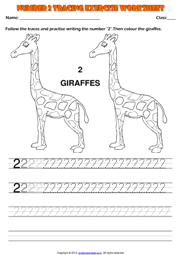A practical printable maths tracing exercise worksheet with lions theme to teach kindergarten, nursery school and preschool kids and students how to write the number 3 (three) and how to count. Look at the samples. Follow the traces and practise writing the number 3. Then color the lions.

#### Number 3 Kindergarten Tracing Exercise Maths Worksheet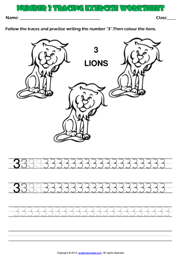##### Number 4 Kindergarten Tracing Exercise Maths Worksheet
An enjoyable printable maths tracing exercise worksheet with pandas theme to teach kindergarten, nursery school and preschool kids and students how to write the number 4 (four) and how to count. Look at the samples. Follow the traces and practise writing the number 4. Then color the pandas.# Kindergarten Numbers Printable Worksheets and Exercises

###### Number 5 Kindergarten Tracing Exercise Maths Worksheet
A useful printable maths tracing exercise worksheet with parrots theme to teach kindergarten, nursery school and preschool kids and students how to write the number 5 (five). Look at the samples. Follow the traces and practise writing the number 5. Then color the parrots.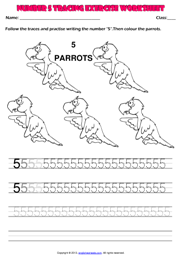## Number 6 Kindergarten Tracing Exercise Maths Worksheet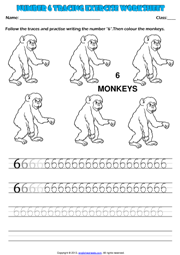A fun printable maths number tracing exercise worksheet with monkeys theme to teach kindergarten, nursery school and preschool kids and students how to write the number 6 (six). Look at the samples. Follow the traces and practise writing the number 6. Then color the monkeys.

### Number 7 Kindergarten Tracing Exercise Maths Worksheet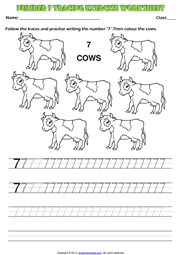A simple printable maths number 2 tracing exercise worksheet with cows theme to teach kindergarten, nursery school and preschool kids and students how to write the number 7 (seven). Look at the samples. Follow the traces and practise writing the number 7. Then color the cows.
##### Number 9 Kindergarten Tracing Exercise Maths Worksheet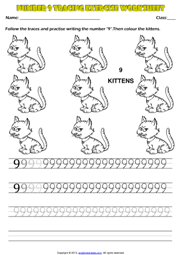An enjoyable printable maths tracing exercise worksheet with kittens theme to teach kindergarten, nursery school and preschool kids and students how to write the number 9 (nine). Look at the samples. Follow the traces and practise writing the number 9. Then color the kittens.

#### Number 8 Kindergarten Tracing Exercise Maths Worksheet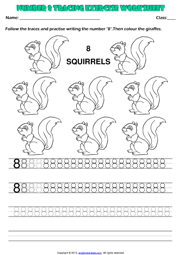A practical printable maths tracing exercise worksheet with squirrels theme to teach kindergarten, nursery school and preschool kids and students how to write the number 8 (eight). Look at the samples. Follow the traces and practise writing the number 8. Then color the squirrels.
###### Number 10 Kindergarten Tracing Exercise Maths Worksheet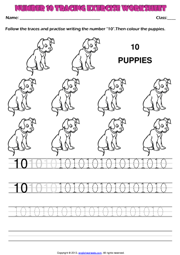A useful printable maths tracing exercise worksheet with puppies theme to teach kindergarten, nursery school and preschool kids and students how to write the number 10 (ten). Look at the samples. Follow the traces and practise writing the number 10. Then color the puppies.

## Numbers 1-9 Kindergarten Writing Exercise Worksheet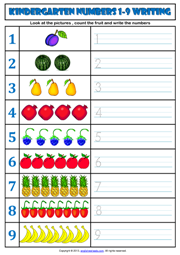A fun printable maths tracing exercise worksheet with fruit theme to teach kindergarten, nursery school and preschool kids and students how to write numbers from 1 to 9. Look at the pictures , count the fruit and write the numbers.